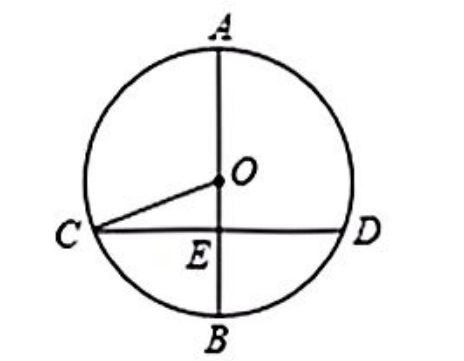$\text{A.}$ $\frac{7}{13}$ $\text{B.}$ $\frac{7}{12}$ $\text{C.}$ $\frac{12}{13}$ $\text{D.}$ $\frac{13}{12}$
【答案】 B